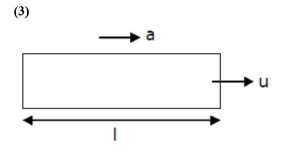# An engine of a train, moving with uniform acceleration,

Question:

An engine of a train, moving with uniform acceleration, passes the signal post with velocity $u$ and the last compartment with velocity v. The velocity with which middle point of the train passes the signal post is :

1. (1) $\sqrt{\frac{v^{2}-u^{2}}{2}}$

2. (2) $\frac{\mathrm{v}-\mathrm{u}}{2}$

3. (3) $\sqrt{\frac{v^{2}+u^{2}}{2}}$

4. (4) $\frac{\mathrm{u}+\mathrm{v}}{2}$

Correct Option: , 3

Solution:$\mathrm{a}=$ uniform acceleration

$\mathbf{u}=$ velocity of first compartment $\mathrm{v}=$ velocity of last compartment $\mid=$ length of train

$v^{2}=u^{2}+2$ as $\left(3^{\text {rd }}\right.$ equation of motion $) \mathrm{v}^{2}=\mathrm{u}^{2}+2 \mathrm{al}$

$\mathrm{v}_{\text {middle }}^{2}=\mathrm{u}^{2}+2 \mathrm{a} \frac{1}{2}$

$\therefore \mathrm{v}_{\text {middle }}^{2}=\mathrm{u}^{2}+\mathrm{al} \quad \ldots(2)$

from equation (1) and (2) $\mathrm{v}_{\text {middle }}^{2}=\mathrm{u}^{2}+\left(\frac{\mathrm{v}^{2}-\mathrm{u}^{2}}{2}\right)$

$=\frac{u^{2}+u^{2}}{2}$

$\therefore \mathrm{v}_{\text {middle }}=\sqrt{\frac{\mathrm{v}^{2}+\mathrm{u}^{2}}{2}}$